# Boolean Algebra Basics

Boolean algebra basics contains a set of axioms, postulates, and theorems. It also contains a set of elements and operators. The operators are called the binary operators.

A set of elements is a collection of objects that have similar properties. This definition of a set is debatable, but that is not the scope of this article. The general notion of set is that it contains elements of similar nature.

Example of Set:

S = \{2, 4, 6, 8 \}

The notation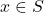mean “element x is in set S”.

The * is a binary operator if for any pair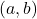it define the rule to find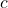and also,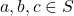. It is not a binary operator is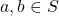and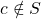.

## Fundamental Postulates of Boolean Algebra

The fundamental postulates for Boolean Algebra are given below. Thought Boolean algebra was introduced by George Boole in 1845, the postulates were formulated by E.V.Huntington in 1904, that every Boolean algebra must satisfy.

We only use two variable Boolean algebra as far as digital design is concerned. The 6 postulates in Boolean algebra basics are listed below.

1. Closure
2. Associative law
3. Commutative law
4. Identity element
5. Inverse
6. Distributive law

Let us discuss each of them, one at a time.

## Closure

A set S is closed with respect to binary operator * if for every pair of an element in set S, the binary operator specifies a rule to find an element in S.

Example,

N is set of natural numbers,

\begin{aligned}
&N = \{1, 2, 3, 4, ...\}
\end{aligned}

Suppose + is the binary operator, then you take any number a and b in N,

\begin{aligned}
&a + b \in N\\
&3 + 2 = 5\\
&2, 3 \in N \\
&5 \in N.\\
\end{aligned}

but when we do subtraction.

\begin{aligned}
&a - b \notin N\\
&2 - 4 = -2\\
&2, 4 \in N \hspace{3px} and \hspace{3px} -2 \notin N\\
\end{aligned}

The subtraction (-) is not a binary operator because it does not satisfy closure property.

## Associativity Law

A binary operator (*) is associative on a set S whenever following is true.

\begin{aligned}&x*(y*z) = (x * y) * z , \\\\
&\forall x, y, z \in S
\end{aligned}

For example, the multiplication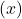is an associative binary operator on a set of natural numbers N.

\begin{aligned}
&= 2 \times ( 4 \times 1)\\
&= 2 \times 4 = 8\\
\end{aligned}

It is equal to the following.

\begin{aligned}
& = (2 \times 4) \times 1\\
& = 8 \times 1 = 8
\end{aligned}

But addition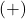is also an associative binary operator.

\begin{aligned}
&= 2 + ( 4 + 5)\\
&= 2 + 9 = 114
\end{aligned}

Is equal to the following equation.

\begin{aligned}
&= (2 + 4) + 5\\
& = 6 + 5 = 11
\end{aligned}

## Communicative Law

A binary operator * is communicative on a set S, whenever

\begin{aligned}
&x * y = y * x, \\\\
&\forall x, y \in S
\end{aligned}

The addition operator (+) and multiplication (x) are communicative on a set of natural numbers (N).

For example,

\begin{aligned}
&5 + 4 = 4 + 5 = 9\\\\
&and\\\\
&4 \times 2 = 2 \times 4 = 8
\end{aligned}

## Identity Element

A set S has an identity element with respect to a binary operation * on S, if there exists an element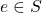such that

\begin{aligned}
&x * e = e * x = x,\\\\
&for \hspace{3px} any \hspace{3px} x \in S
\end{aligned}

The identity element for a set S is always unique to the set. Consider the following example, where + is the binary operation on a set of integers, Z and 0 is the identity element.

\begin{aligned}
Z = 4 + 0 = 0 + 4 = 4\\
Z = 8 + 0 = 0 + 8 = 8
\end{aligned}

## Inverse

A set S with identity element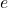is said to have an inverse with respect to binary operation *, whenever, for all

\begin{aligned}
&x \in S,\\
&\exists y \in S \mid x * y = e\end{aligned}

Let us take the previous example of addition + on a set of integers Z, with identity element 0.

Let x be an element in Z,

\begin{aligned}
&x \in Z\\
&x = 5\\
\end{aligned}

Then, -x also belong to Z, let us call it y.

\begin{aligned}
&y \in Z\\
&y = -5\\
&x + y = 0
\end{aligned}

Therefore, a set of integers have inverse with respect to binary operation – addition.

## Distributive Law

The distributive law is a very important property of algebraic structures. If * and. are two binary operations on set S, then * is said to distributive over. whenever

\begin{aligned}
&x * ( y . z) = (x * y) . (x * z)\\\\
&\forall x,y,z \in S
\end{aligned}

Also, . is distributive over * whenever

\begin{aligned}
&x \cdot (y \cdot z) = (x \cdot y) \cdot (x \cdot z)\\\\
&\forall x, y, z \in S
\end{aligned}

From the above postulate in Boolean algebra basics, it clear that all binary operators cannot hold all six properties. In fact, the modern algebra studies these algebraic structures formally and trying to find interesting properties. The topics of study are groups, sub-groups, monoid, rings, and fields.